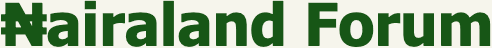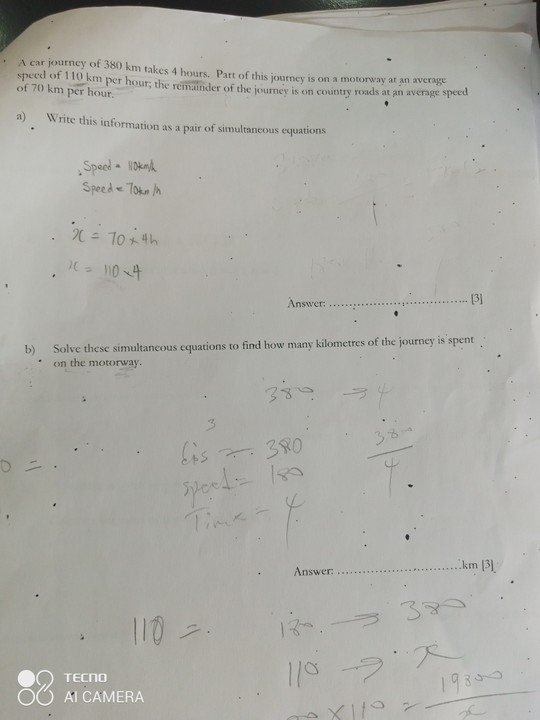#Welcome, Guest: Join Nairaland / LOGIN! / Trending / Recent / New
Stats: 2,756,068 members, 6,548,334 topics. Date: Thursday, 21 October 2021 at 01:14 AM

## First Correct Answer Get 1000 Naira Airtime - Family - Nairaland

 First Correct Answer Get 1000 Naira Airtime by pimpchi(m): 4:15pm On Mar 06 Solve and show workingsRe: First Correct Answer Get 1000 Naira Airtime by shamsin6300(m): 4:21pm On Mar 06 I will need part of the money to buy pen and exercise book to solve this down. Re: First Correct Answer Get 1000 Naira Airtime by dejt4u(m): 5:31pm On Mar 06 pimpchi:Solve and show workings (a) Given:Speed for the journey = 380 kmTotal time taken for the journey = 4hoursThe journey was in two parts. First part of the journey was on the motorway and the second part was on the country roadLet x and y represents the distance and time taken respectively for the journey in the first part (on the motorway);Therefore, the speed on the motorway is calculated as x ÷ y = 110 km/hx = 110y ...... Eqn 1To get equation 2, let's find the expression for speed on the country roadDistance on the country road = 380km - xTime spent on the country road = 4hrs - yTherefore, the speed on the country road = (380 - x) / (4- y) = 70km/h,380 - x = 70(4 -y),380 - x = 280 - 70y,380 - 280 = x - 70y,x - 70y = 100 ....Eqn 2Therefore, these are the simultaneous equations x = 110y ...Eqn 1x - 70y = 100 ...Eqn 2(b) Solving the equations;Sub for x in eqn 2 using eqn 1,x - 70y = 100,110y - 70y = 10040y = 100y = 100/40 = 2.5 hourssub for y in eqn 1,x = 110y,x = 110 (2.5),x = 275kmTherefore the distance covered on the motorway is 275km@pimpchiFeel free to ask questions. Re: First Correct Answer Get 1000 Naira Airtime by Chapter1vs6(m): 5:42pm On Mar 06 I couldn't even get past speed 110km/hRe: First Correct Answer Get 1000 Naira Airtime by pimpchi(m): 6:33pm On Mar 06 dejt4u:(a) Given:Speed for the journey = 380 kmTotal time taken for the journey = 4hoursThe journey was in two parts. First part of the journey was on the motorway and the second part was on the country roadLet x and y represents the distance and time taken respectively for the journey in the first part (on the motorway);Therefore, the speed on the motorway is calculated as x ÷ y = 110 km/hx = 110y ...... Eqn 1To get equation 2, let's find the expression for speed on the country roadDistance on the country road = 380km - xTime spent on the country road = 4hrs - yTherefore, the speed on the country road = (380 - x) / (4- y) = 70km/h,380 - x = 70(4 -y),380 - x = 280 - 70y,380 - 280 = x - 70y,x - 70y = 100 ....Eqn 2Therefore, these are the simultaneous equations x = 110y ...Eqn 1x - 70y = 100 ...Eqn 2(b) Solving the equations;Sub for x in eqn 2 using eqn 1,x - 70y = 100,110y - 70y = 10040y = 100y = 100/40 = 2.5 hourssub for y in eqn 1,x = 110y,x = 110 (2.5),x = 275kmTherefore the distance covered on the motorway is 275km@pimpchiFeel free to ask questions. Wrong Re: First Correct Answer Get 1000 Naira Airtime by Nobody: 10:11pm On Mar 06 pimpchi:Wrong He's right. To simplify the solution, if you work out 380= 110y + 70(4-y); where y = amount of time spent on the motorway, you get 275km (2.5hours × 110km/h) on the motorway and 105km (1.5hours × 70km/h on the country road); which is what he gave. His derivation of the two equations is also correct. Re: First Correct Answer Get 1000 Naira Airtime by dejt4u(m): 10:46pm On Mar 06 pimpchi:Wrong lol. the solution is what I have provided for you